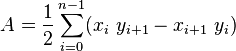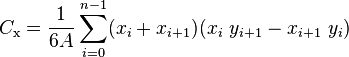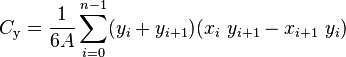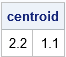Recently I blogged about how to compute a weighted mean and showed that you can use a weighted mean to compute the center of mass for a system of N point masses in the plane. That led me to think about a related problem: computing the center of mass (called the centroid) for a planar polygon.

If you cut a convex polygon out of stiff cardboard, the centroid is the position where the polygon would balance on the tip of a needle. However, for non-convex polygons, the centroid might not be located in the interior of the polygon.

For a general planar figure, the mathematical formula for computing the centroid requires computing an integral. However, for polygons there are some well-known equations that involve only the locations of the vertices (provided that the vertices are enumerated in a consistent clockwise or counter-clockwise manner). The Wikipedia article about the centroid give a useful computational formula. It turns out that the (signed) area of a simple polygon with vertices (x0, y0), (x1, y1), ..., (xN-1, yN-1), is given byIn the formula, the vertex (xN, yN) is identified with the first vertex, (x0, y0). The centroid of the polygon occurs at the point (Cx, Cy), whereA polygon whose vertices are enumerated in a counter-clockwise manner will have a positive value for A. The formula for A will be negative when vertices are enumerated in a clockwise manner.

In the SAS language, arrays use 1-based indexing by convention. Therefore, when implementing the formula in SAS, it is customary for the vertices to be enumerated from 1 to N. The limits in the summation formulas are modified similarly. The (N+1)st vertex is identified with the first vertex.

Centroids are sometimes used as a position to label a country or state on a map. For SAS customers who have a license for SAS/GRAPH, the official SAS %CENTROID macro computes the centroid of a polygonal region. It is designed for use with map data sets, which have variables named ID, X, and Y. My colleague Sanjay Matange provides a similar macro in his blog post "A Macro for Polygon Area and Center."

### A vectorized SAS/IML function that computes a centroid

Implementing the centroid formulas in SAS/IML is a good exercise in vectorization. Recall that function is vectorized if it avoids unnecessary loops and instead uses vector and matrix operations to perform the computations. For the area and centroid formulas, you can form a vector that contains all of the values that are to be summed. You can use the elementwise multiplication operator (#) to compute the elementwise product of two vectors. The SUM function adds up all elements in a vector.

The following function computes the centroid of a single polygon in a vectorized manner. The first few statements build the vectors from the values of the vertices; the last four statements compute the area and centroid by using vectorized operations. There are no loops.

```proc iml; /* _PolyCentroid: Return the centroid of a simple polygon. P is an N x 2 matrix of (x,y) values for consectutive vertices. N > 2. */ start _PolyCentroid(P); lagIdx = 2:nrow(P) || 1; /* vertices 2, 3, ..., N, 1 */ xi = P[ ,1]; yi = P[ ,2]; /* x_i and y_i for i=1..N */ xip1 = xi[lagIdx]; yip1 = yi[lagIdx]; /* x_{i+1} and y_{i+1}, x_{N+1}=x_1 */ d = xi#yip1 - xip1#yi; /* vector of difference of products */ A = 0.5 * sum(d); /* signed area of polygon */ cx = sum((xi + xip1) # d) / (6*A); /* X coord of centroid */ cy = sum((yi + yip1) # d) / (6*A); /* Y coord of centroid */ return( cx || cy ); finish;   /* test the function by using an L-shaped polygon */ L = {0 0, 6 0, 6 1, 2 1, 2 3, 0 3}; centroid = _PolyCentroid(L); print centroid;```For this L-shaped polygon, the centroid is located outside the polygon. This example shows that the centroid might not be the best position for a label for a polygon. Examples of this phenomenon are easy to find on real maps. For example, the states of Louisiana and Florida have centroids that are near the boundaries of the states.

### Compute the centroid of many polygons

The %CENTROID macro can compute the centroids for multiple polygons, assuming that there is an identifying categorical variable (called the ID variable) whose unique values distinguish one polygon from another.

If you want to compute the centroids of multiple polygons in SAS/IML, you can use the UNIQUEBY function to extract the rows that correspond to each polygon. For each polygon, the previous _PolyCentroid function is called, as follows:

```/* If the polygon P has two columns, return the centroid. If it has three columns, assume that the third column is an ID variable that identifies distinct polygons. Return the centroids of the multiple polygons. */ start PolyCentroid(P); if ncol(P)=2 then return( _PolyCentroid(P) ); ID = P[,3]; u = uniqueby(ID); /* starting index for each group */ result = j(nrow(u), 2); /* allocate vector to hold results */ u = u // (nrow(ID)+1); /* append (N+1) to end of indices */ do i = 1 to nrow(u)-1; /* for each group... */ idx = u[i]:(u[i+1]-1); /* get rows in group */ result[i,] = _PolyCentroid( P[idx, 1:2] ); end; return( result ); finish;   /* test it on an example */ rect = {0 0, 2 0, 2 1, 0 1}; ID = j(nrow(L), 1, 1) // j(nrow(rect), 1, 2); P = (L // rect) || ID; centroids = PolyCentroid(P); print centroids;```In summary, you can construct an efficient function in SAS/IML to compute the centroid of a simple polygon. The construction shows how to vectorize a computation by putting the vertices in vectors, rather than the less efficient alternative, which is to iterate over the vertices in a loop. Because polygons are often used to display geographical regions, I also included a function that iterates over polygons and computes the centroid of each.

tags: Math, vectorization

The post Compute the centroid of a polygon in SAS appeared first on The DO Loop.